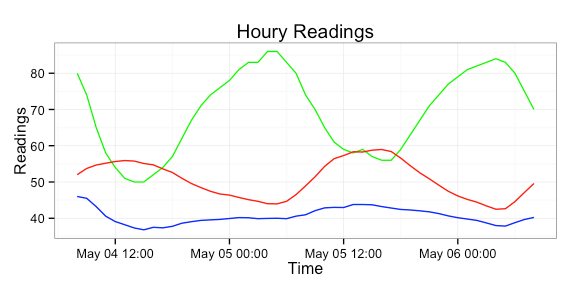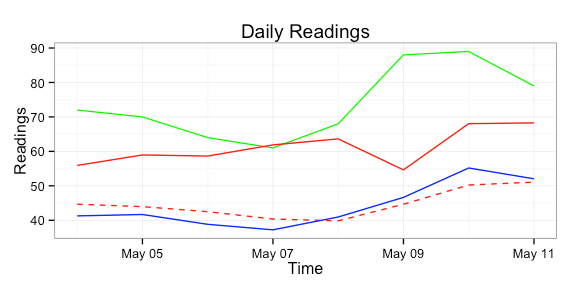# Rforecastio Package Update (1.1.0)

I’ve bumped up the version number of `Rforecastio` ([github](https://github.com/hrbrmstr/Rforecastio)) to `1.1.0`. The new
features are:

– removing the SSL certificate bypass check (it doesn’t need it
anymore)
– using `plyr` for easier conversion of JSON-\>data frame
– adding in a new `daily` forecast data frame
– roxygen2 inline documentation

```library(Rforecastio)
library(ggplot2)
library(plyr)

# NEVER put API keys in revision control systems or source code!

my.latitude = "43.2673"
my.longitude = "-70.8618"

fio.list <- fio.forecast(fio.api.key, my.latitude, my.longitude)

fio.gg <- ggplot(data=fio.list\$hourly.df, aes(x=time, y=temperature))
fio.gg <- fio.gg + geom_line(aes(y=humidity*100), color="green")
fio.gg <- fio.gg + geom_line(aes(y=temperature), color="red")
fio.gg <- fio.gg + geom_line(aes(y=dewPoint), color="blue")
fio.gg <- fio.gg + theme_bw()
fio.gg``````fio.gg <- ggplot(data=fio.list\$daily.df, aes(x=time, y=temperature))
fio.gg <- fio.gg + geom_line(aes(y=humidity*100), color="green")
fio.gg <- fio.gg + geom_line(aes(y=temperatureMax), color="red")
fio.gg <- fio.gg + geom_line(aes(y=temperatureMin), color="red", linetype=2)
fio.gg <- fio.gg + geom_line(aes(y=dewPoint), color="blue")
fio.gg <- fio.gg + theme_bw()
fio.gg```Amazon Author Page

## 7 Comments →Rforecastio Package Update (1.1.0)

1.Stefan

Hi,

first time I stumbled upon your package so I’m sorry if I ask stupid questions. I’m using the Rforecastio version from 4 hours ago on R 3.1.0

1. I don’t know what the “SSL certificate bypass check” did but something is needed:

Error in function (type, msg, asError = TRUE) :
SSL certificate problem, verify that the CA cert is OK. Details:
error:14090086:SSL routines:SSL3GETSERVER_CERTIFICATE:certificate verify failed

1. When I set ssl.verifypeer=FALSE I get a:

Error in as.POSIXlt.default(fio.daily.df\$precipIntensityMaxTime, origin = “1970-01-01”) :
do not know how to convert ‘fio.daily.df\$precipIntensityMaxTime’ to class “POSIXlt”

This seems to happen because precipIntensityMaxTime doesn’t exist and as.POSIXlt doesn’t like to convert NULL.

Otherwise this package is a great idea. I hope to see it on CRAN soon.

2.Stefan

The following at the beginning of fio.forecast solved the second issue – a cleaner solution would be a different function name, of course:

as.POSIXlt<-function(x,…)
if(!is.null(x)) base::as.POSIXlt(x,…) else NULL

1.hrbrmstr

thx. i added a `...` option in the function to enable passing in of CURL options (so you can use ssl.verifypeer=FALSE and even set proxies if you need it). I also did the NULL test and fixed another issue. Thx for checking out the package & giving me a heads up on the issues!

3.dksam

Sir, please how can I download yearly / 5 / 10 yearly data, I need it for Disease forecasting
How can i plot more information like day temperature, night temperature, wind speed etc,
thanks for such a wonderful piece of software, Dr.D.K.Samuel, Sr.Scientist, IIHR, Bangalore – 89, India

1.hrbrmstr

After taking a quick look at the forecast.io API, I think there’s more efficacy in using the wunderground or NCDC historical weather data. This blog has help on getting such data, including [this comment](http://www.ncdc.noaa.gov/cdo-web/search?datasetid=GHCND) on that post.

4.dksam

Samuel

fio.list <- fio.forecast(fio.api.key, my.latitude, my.longitude)
Error in function (type, msg, asError = TRUE) :
SSL certificate problem, verify that the CA cert is OK. Details:
error:14090086:SSL routines:SSL3GETSERVERCERTIFICATE:certificate verify failed
fio.gg <- ggplot(data=fio.list\$hourly.df, aes(x=time, y=temperature))
Error in ggplot(data = fio.list\$hourly.df, aes(x = time, y = temperature)) :
fio.gg <- fio.gg + geom
line(aes(y=humidity100), color=”green”)
fio.gg <- fio.gg + geom
line(aes(y=temperature), color=”red”)
fio.gg <- fio.gg + geom
line(aes(y=dewPoint), color=”blue”)
fio.gg <- fio.gg + themebw()
fio.gg
fio.list <- fio.forecast (fio.api.key, my.latitude, my.longitude, proxy=’host:port’ and ssl.verifypeer=FALSE)
Error: unexpected symbol in “fio.list <- fio.forecast (fio.api.key, my.latitude, my.longitude, proxy=’host:port’ and”
fio.gg <- ggplot(data=fio.list\$hourly.df, aes(x=time, y=temperature))
Error in ggplot(data = fio.list\$hourly.df, aes(x = time, y = temperature)) :
fio.gg <- fio.gg + geom
line(aes(y=humidity100), color=”green”)
fio.gg <- fio.gg + geomline(aes(y=temperature), color=”red”)
1.hrbrmstr
Please see the 1.2.0 update on github. You’ll need to specify `ssl.verifypeer=FALSE` in the parameter list. I’ll see if I can get to adding a bulk downloading later this week.